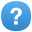# DecimalFormat does not allow formatting BigDecimal with more than 340 or 309 digits

## Description

The DecimalFormat class does not allow BigDecimal numbers to be formatted with more than 340 fractional digits or 309 integer digits. This makes for a very limited BigDecimal class.

The DecimalFormat.setMaximumFractionDigits method silently caps the number of digits after the decimal point to 340, although this breaks the usual contract of java.text.DecimalFormat, which states that that method "Sets the maximum number of digits allowed in the fraction portion of a number. For formatting numbers other than BigInteger and BigDecimal objects, the lower of newValue and 340 is used."

However, the ICU implementation erroneously limits all number types, including BigDecimal, to 340 digits after the decimal point. The fix was probably intended to only affect the Java "double" type, but the bug affects BigDecimal so it is impossible to use BigDecimal as intended and produce an arbitrary number of digits.

The DecimalFormat.setMaximumIntegerDigits method similarly caps the number of digits before the decimal point to 309. This breaks the usual contract of java.text.DecimalFormat, which states that the method "Sets the maximum number of digits allowed in the integer portion of a number. For formatting numbers other than BigInteger and BigDecimal objects, the lower of newValue and 309 is used."

However, the ICU implementation erroneously limits all number types, including BigDecimal, to 309 digits before the decimal point. The fix was probably intended to only affect the Java "double" type, but the bug affects BigDecimal so it is impossible to use BigDecimal as intended and produce an arbitrary number of digits.

TracBot

TracBot

None

None

## Priorityassess

None

None
Configure